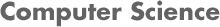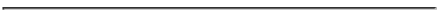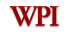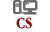### Miscellaneous Notes on Parametric Machine Learning Methods in Matlab#### Generating Normally Distributed Random Data:

Generate a set X of 100 random numbers following a normal distribution. In this example: the normal distribution for X has mean 90 and standard deviation 10.
```>> X = random('Normal',90,10,1,100);
```

#### Maximum Likelihood Estimation (MLE):

Perform Maximum Likelihood Estimation (mle matlab function) on the set X generated above:
```>> T = mle(X);
>> T

T =

91.2309   11.5657
```
This mean=91.2309 and standard deviation=11.5657 come from estimating maximum likelihood using the equations (4.8) on p. 68 of Alpaydin's textbook (3rd edition):
```mean = sum(x^t)/N
sd^2 = sum (x^t - mean)^2 / N
```
where N = 100 in this example.
```>> m = sum(X)/100

m =

91.2309

>> s = sqrt(sum((X - [sum(X)/100]).^2)/100)

s =

11.5657
```
Note that [T,pci] = mle(___) also returns the 95% confidence intervals for the parameters:
```>> [T,pci] = mle(X)

T =

91.2309   11.5657

pci =

88.9244   10.2059
93.5373   13.5033

```
Note also that as the sample size increases, the maximum likelihood estimations get closer to the actual parameter values:
```>> X2 = random('Normal',90,10,1,1000);
>> mle(X2)

ans =

89.6348    9.8753

>> X3 = random('Normal',90,10,1,10000);
>> mle(X3)

ans =

90.0433    9.8635
```
We can see the histogram plots for each of these random sets:
```>> figure; hist(X)
>> figure; hist(X2)
>> figure; hist(X3)
```
Note: Matlab's mle function can also calculate mle on non-Gaussian distributions.

#### Generating Normally Distributed Random Data to Illustrate Parametric Classification:

Generate two sets X and Y of 100 random numbers, where each set following a normal distribution. In this example:
• the normal distribution for X has mean 90 and standard deviation 10.
• the normal distribution for Y has mean 60 and standard deviation 10.
Below is the Matlab program two_random_normal_sets.m that I used to achieve this.
```C1 = random('Normal',60,10,1,100);
C2 = random('Normal',90,5,1,100);
D(1:100,1) = C1;
D(1:100,2) = 1;
D(1:100,3) = 0;
D(101:200,1) = C2;
D(101:200,2) = 0;
D(101:200,3) = 1;
```
D contains now 200 data instances, whose first column is a randomly generated number, and its second and third columns tell if the number came from C1 or from C2.

We can use histograms to plot C1 and C2:

```>> figure; hist(C1)
>> figure; hist(C2)
```

#### Parametric Classification:

We can use equations (4.25) and (4.26) on p. 74 of Alpaydin's textbook (3rd edition):
```(4.25) m_i = sum_t(x^t * r^t_i)/N_i
(4.26) s^2_i = sum_t (x^t - m_i)^2 * r^t_i/ N_i

where: N_i = sum_t(r^t_i)
```
```
>> N1= sum(D(:,2))

N1 =

100

>> N2= sum(D(:,3))

N2 =

100

>> m1 = sum(D(:,1).*D(:,2))/N1

m1 =

60.9839

>> m2 = sum(D(:,1).*D(:,3))/N2

m2 =

90.1958

>> s1 = sqrt(sum(D(:,2).*(D(:,1)- m1).^2)/N1)

s1 =

8.9451

>> s2 = sqrt(sum(D(:,3).*(D(:,1)- m2).^2)/N2)

s2 =

4.5562
```
Now using equation (4.28) p. 75 after disregarding its first term (which is constant) and its forth term (assuming the prior probabilities of C1 and C2, P(C1) and P(C2) are the same), we get:
```g_i(x) = - log s_i - ((x - m_i)^2/(2 s_i^2))
```
```
g1 = @(x) - log(s1) - ((x - m1)^2/(2*s1^2))

g2 = @(x) - log(s2) - ((x - m2)^2/(2*s2^2))

```
In order to classify a data instance x, we calculate g1(x) and g2(x), and pick the class corresponding to the larger of these two values. That is,
```ChooseCi(x): If g1(x) >= g2(x) then pick C1 else pick C2
```
For example, for x=50:
```>> if g1(50) >= g2(50)
1
else
2
end

ans =

1

```ruiz@cs.wpi.edu